# Beginning Algebra Review Exam 4## Material covered: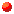Reducing and Building Fractions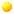Adding and Subtraction Fractions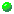Multiplying and Dividing Fractions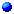Complex Fractions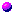Solving Fractional Equations
```
1.  Write in standard form.

a.  - (-7/2)     b.  (x - 3)/(-2)    c.  - ( (2-y)/3 )

2.  Reduce.

a.  16/28     b.  32a³ b/36a² b³     c.  (4x²  + 20x + 25)/(4x² - 25)

3.  Express as an equivalent fraction with indicated denominator.

a.  (x - 1)/(x + 2) = ?/(x²  - 4)     b.  (x + 7)/(x - 2) = ?/(x²  + x - 6)

4.  Perform the indicated operations.  Be sure to reduce final answer to lowest terms.

a.  (2x)/( 5(x + 3) ) + 6/( 5(x + 3) )

b.  x/(x²  - 9) - 3/(x²  - 9)

c.  (4x)/(3y² ) + x/(6y)

d.  (5x)/(x²  + 8x + 16) - (3x)/(x²  + 6x + 8)

e.  (2x - 1)/(x²  + 7x + 10) - (x + 1)/(x²  + 8x + 15)

f.  (4a² )/(9b² c)(6bc² )/(5a)

g.  ( (15a³ b)/(7c) ) / ( (5a) /(14c² ) )

h.  ( (2x + 14)/(x²  + 2x - 15) )/( (x²  + 8x + 7)/(x²  - 2x - 3) )

i.  ( (2x - 10)/(x²  - 1) )( ( x²  + 6x + 5)/(4x² ) )( (4)/(x² - 25) )

5.  Write as a simple fraction in lowest terms.  The main fraction bar is the one in bold face type.

a.  (2/3)/(7/5)     b.  ((4x)/3)/((2x)/5)     c.  (x - 1/x)/(x + 1/x)

6.  Use long division technique to divide each of the following.

a.  (2x³  + x²  - 5)/(x + 3)     b.  (2x²  - 3x + 4)/(x - 2)

7.  Solve for x.

a.  (5x²)/8 - (3x)/4 = 1     b.  4/(x - 4) + 3/(x + 1) = 20/(x²  - 3x - 4)

8.  Solve each of the following stated problems.

a.  Antoine traveled 36 miles downstream by motorboat to visit his parents and then returned
home.  Antoine spent 5 hours traveling, and the speed of the current is 3 miles per hour.
How fast does the motorboat travel in still water?

b.  It takes Professor Gilbert 3 hours to grade a set of homework papers, and it takes her
teaching assistant 5 hours to grade a set.  If they work together, how long will it take
them to grade a set of homework papers?

```